# Principled Hair BSDF¶Principled Hair BSDF under Melanin concentration.

Cycles Only

The Principled Hair BSDF is a physically-based, easy-to-use shader for rendering hair and fur.

Astuce

Realistic hair should have a minimum of variance between each strand. The shader allows for this by specifying two values, Random Color and Random Roughness, which remap the specified Melanin/Roughness values to the range $$Color/Roughness \pm Randomization\%$$.

## Inputs¶

Color

The RGB color of the strand. Only used in Direct coloring.

Indication

The chosen color is converted to an absorption coefficient with the following formula (section 4.2 of [CBTB16]):

$\sigma_{a} = \frac{\ln(Color)} {\left(5.969 - 0.215\beta_{N} + 2.532\beta_{N}^{2} - 10.73\beta_{N}^{3} + 5.574\beta_{N}^{4} + 0.245\beta_{N}^{5}\right)^{2}}$

where $$\beta_{N}$$ is the radial roughness of the hair after applying randomization (if specified).Coloring hair using the Direct coloring parametrization. (The numbers on top are the RGB values.)

Melanin

Absolute quantity of pigment. Range $$[0, 1]$$ equivalent to $$[0\%, 100\%]$$.

Indication

This is a linear mapping to the underlying exponential function:

$melanin\_qty = -\ln(\max(1.0 - Melanin, 0.0001))$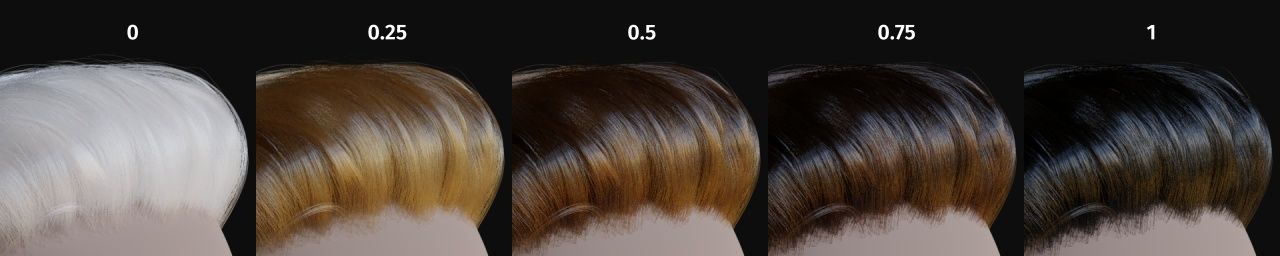Melanin.

Melanin Redness

Ratio of pheomelanin to eumelanin. Range $$[0, 1]$$ equivalent to $$[0\%, 100\%]$$.

Indication

The ratio formula is: $$eumelanin = Melanin*(1.0-MelaninRedness)$$, $$pheomelanin = Melanin*MelaninRedness$$.

The resulting quantities are converted (after randomization, if specified) to absorption concentration via the following formula (section 6.1 of [EFHLA11], adjusted for the range $$[0, 1]$$):

$\begin{split}\sigma_{a} = eumelanin * \left[\begin{matrix} 0.506 \\ 0.841 \\ 1.653 \\ \end{matrix}\right] + pheomelanin * \left[\begin{matrix} 0.343 \\ 0.733 \\ 1.924 \\ \end{matrix}\right]\end{split}$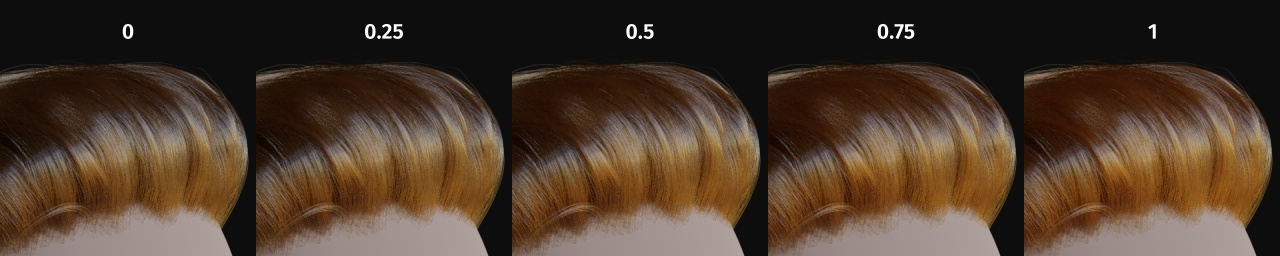Melanin Redness.

Tint

Color used for dyeing the hair after applying the melanin pigment. It is not subject to randomization. It can be disabled by setting the color to white.

Indication

This is converted via the Color mapping above and added to the absorption coefficient of the melanin concentration.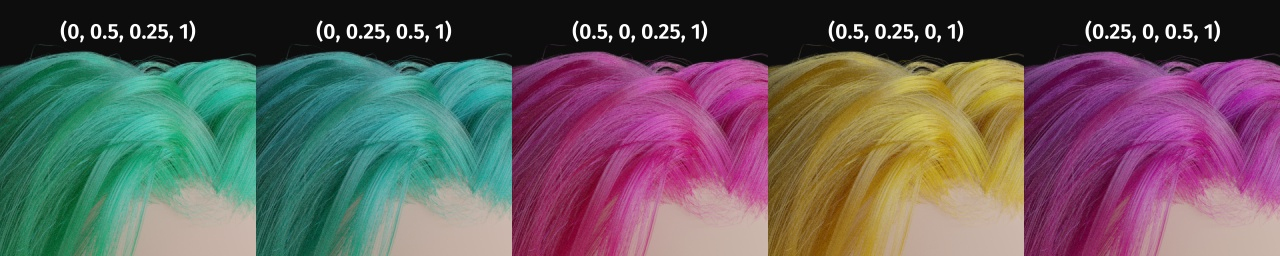Tint, using Melanin 0.1 and the corresponding RGB values.

Absorption coefficient

Attenuation coefficient $$\sigma$$.

Roughness

Specify how much the glints are smoothed in the direction of the hair shaft. Too low values will smoothen the hair to the point of looking almost metallic, making glints look like Fireflies; while setting it too high will result in a Lambertian look.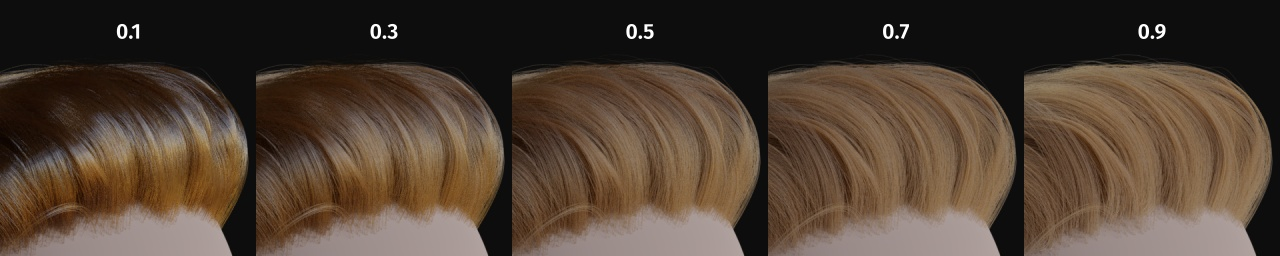Roughness.

Specify how much the glints are smoothed in the direction of the hair tangent. Too low values will concentrate the glint; while setting it too high will spread the light across the width of the strand.

Indication

Mathematically, this parameter is mapped to the logistic distribution’s scale factor $$s$$ (section 4.1 of [CBTB16]).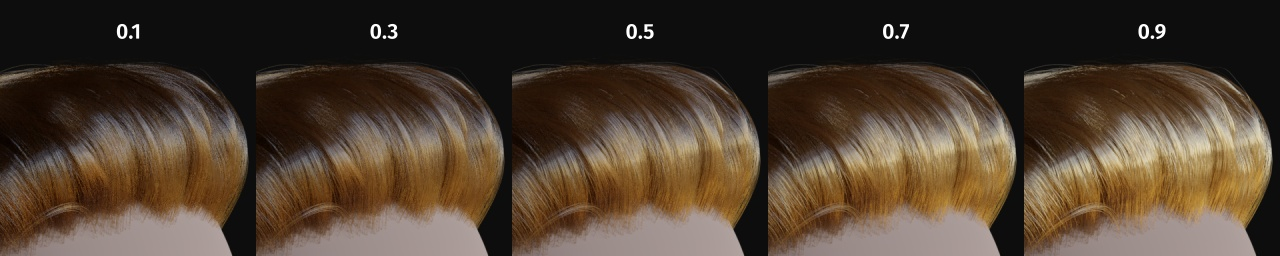Coat

Simulate a shiny coat of fur, by reducing the Roughness to the given factor only for the first light bounce (diffuse). Range $$[0, 1]$$ equivalent to a reduction of $$[0\%, 100\%]$$ of the original Roughness.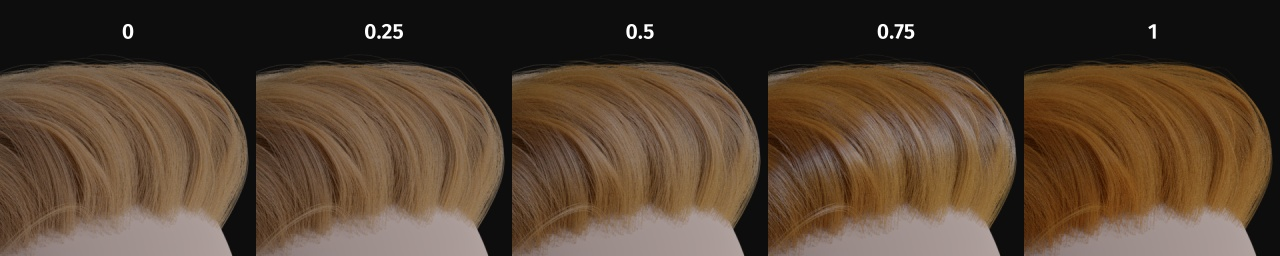Coat.

IOR

Index of refraction (IOR) defining how much the ray changes direction. At 1.0 rays pass straight through like in a transparent material; higher values give more refraction. Default value is $$1.55$$.

Offset

Tilts the glint of the hair by increasing the angle of the scales of the hair’s cuticle with respect to the hair shaft. Human hair usually has low values.

Random Color

For each strand, vary the melanin concentration by $$RandomFactor$$. Range $$[0, 1]$$ equivalent to $$[0\%, 100\%]$$ of the initial melanin concentration.

Indication

The melanin concentration is multiplied by $$randomFactor$$, where $$randomFactor = 1.0 + 2.0*(Random - 0.5) * RandomColor$$.Random Color.

Random Roughness

For each strand, vary both Roughness values by $$RandomFactor$$. Range $$[0, 1]$$ equivalent to $$[0\%, 100\%]$$ of the initial roughness values.

Indication

The applied formula is the same one as for Random Color.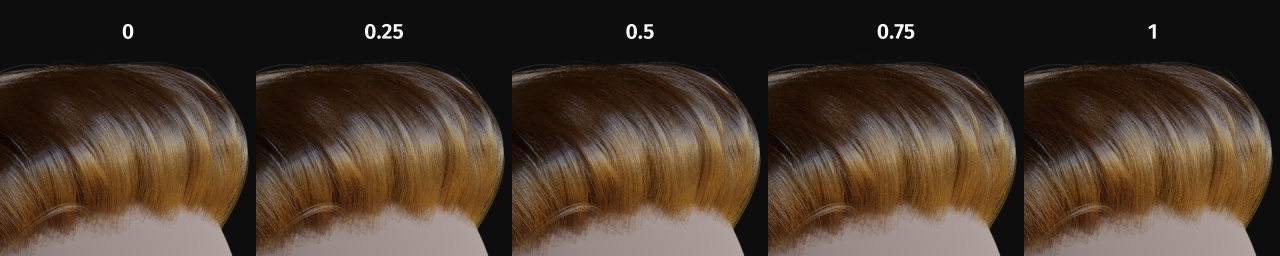Random Roughness.

Random

Random number source. If no node is connected here, it is automatically instanced with the value obtained from Hair Info ‣ Random.

## Properties¶

Color Parametrization

The shader provides three different ways, or parametrizations, to color the hair strands.

Direct coloring

Choose the desired RGB color and the shader will approximate the necessary absorption coefficient (below).

Melanin concentration

This mode defines the color as the quantity and ratio of the pigments which are commonly found in hair and fur, eumelanin (prevalent in brown-black hair) and pheomelanin (red hair). The quantity is specified in the Melanin input, and the ratio between them in Melanin Redness. Increasing concentrations darken the hair (the following are with Melanin Redness $$1$$):

• White (Melanin $$0$$)

• Blonde (Melanin $$0.25$$)

• Reddish (Melanin $$0.5$$)

• Brown (Melanin $$0.75$$)

• Black (Melanin $$1$$)

Additionally, the Tint inputs allows to dye the hair with the desired color.

Absorption coefficient

Specifies the attenuation coefficient $$\sigma_{a}$$, as applied by the Beer-Lambert law. This mode is intended mainly for technical users who want to use coefficients from the literature without any sort of conversion.

BSDF